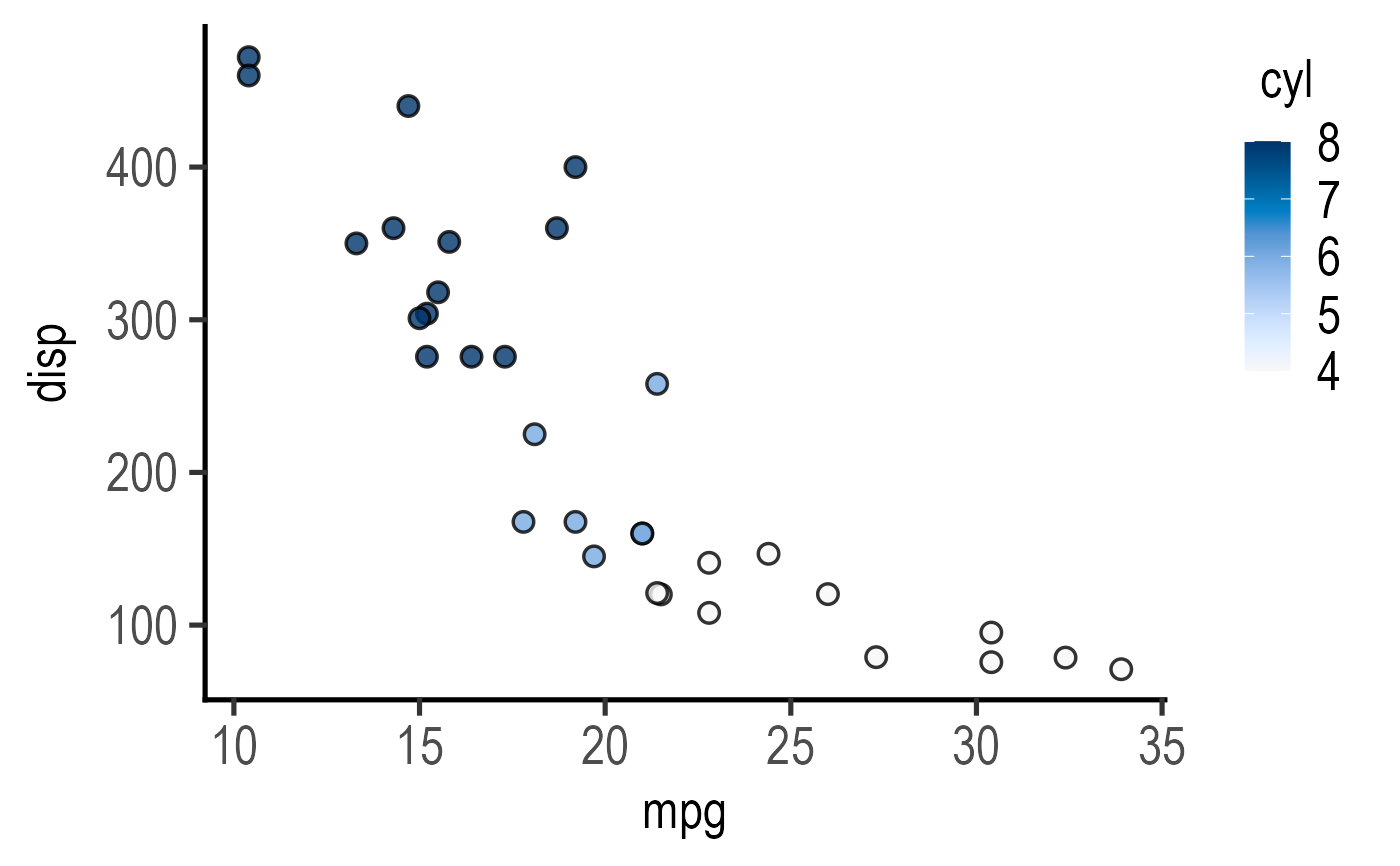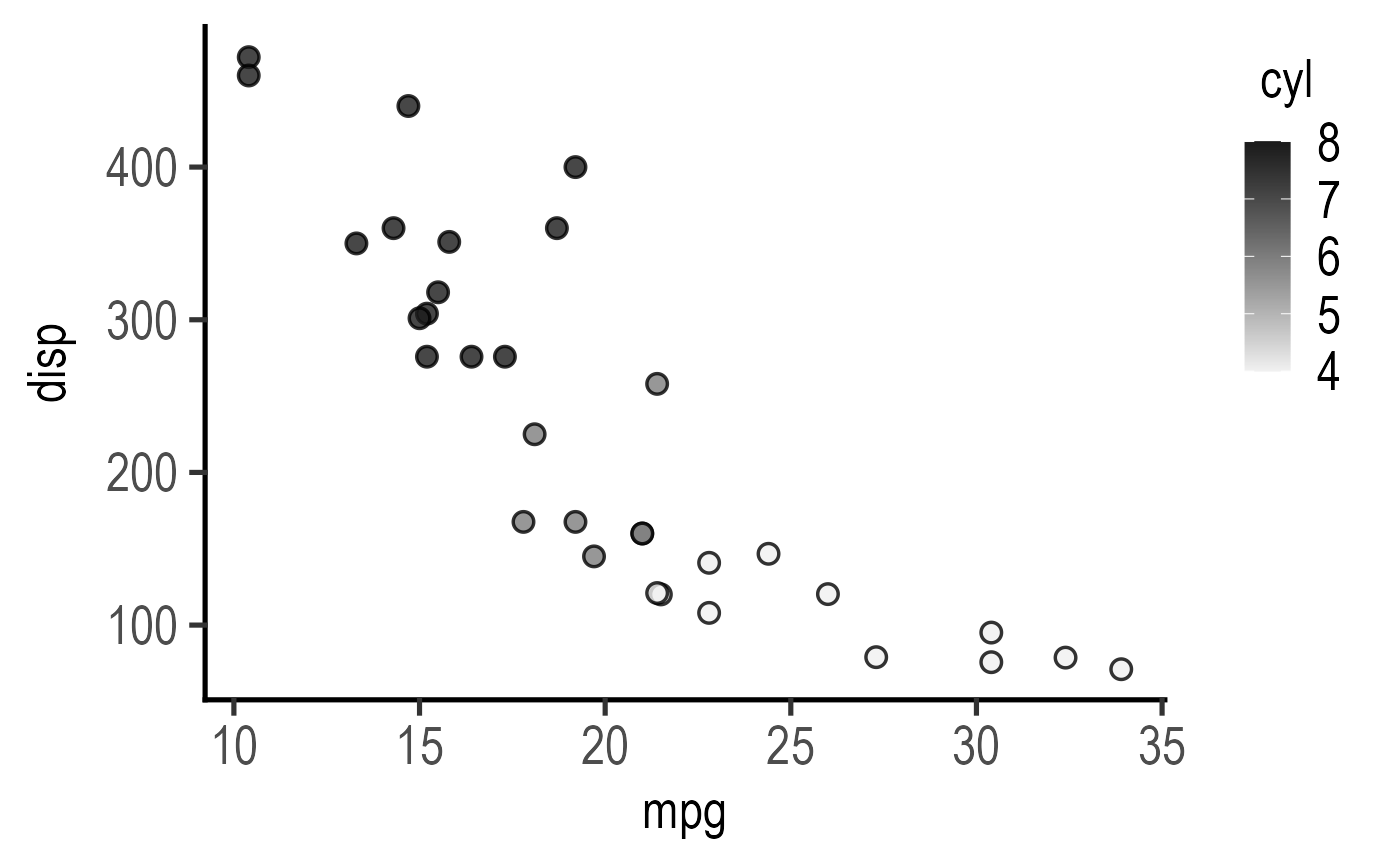This function takes a data table, quantitative X and Y variables, and a numeric grouping variable, and a and plots a graph with using geom_point. The numerical NumGroup variable is mapped to the fill aesthetic of symbols, which receives the scale_fill_grafify default quantitative palette (blue_conti). Alternatives are yellow_conti, grey_conti, OrBl_div and PrGn_div. Colour order can be reversed with ColRev = TRUE (default is FALSE).

plot_xy_NumGroup(
data,
xcol,
ycol,
NumGroup,
facet,
Boxplot = FALSE,
symsize = 3,
s_alpha = 0.8,
TextXAngle = 0,
LogYTrans,
LogXTrans,
LogYBreaks = waiver(),
LogXBreaks = waiver(),
LogYLabels = waiver(),
LogXLabels = waiver(),
LogYLimits = NULL,
LogXLimits = NULL,
facet_scales = "fixed",
fontsize = 20,
bwid = 0.3,
b_alpha = 0.3,
l_alpha = 0.8,
symthick,
bthick,
ColPal = c("blue_conti", "yellow_conti", "grey_conti", "PrGn_div", "OrBl_div"),
ColRev = FALSE,
...
)

## Arguments

data

a data table object, e.g. data.frame or tibble.

xcol

name of the column with quantitative X variable.

ycol

name of the column with quantitative Y variable.

NumGroup

a numeric factor for fill aesthetic of data points.

facet

add another variable from the data table to create faceted graphs using ggplot2facet_wrap.

Boxplot

logical TRUE/FALSE to plot box and whiskers plot (default = FALSE).

symsize

size of symbols used by geom_point. Default set to 3.

s_alpha

fractional opacity of symbols, default set to 0.8 (i.e, 80% opacity).

TextXAngle

orientation of text on X-axis; default 0 degrees. Change to 45 or 90 to remove overlapping text.

LogYTrans

transform Y axis into "log10" or "log2"

LogXTrans

transform X axis into "log10" or "log2"

LogYBreaks

argument for ggplot2[scale_y_continuous] for Y axis breaks on log scales, default is waiver(), or provide a vector of desired breaks.

LogXBreaks

argument for ggplot2[scale_x_continuous] for Y axis breaks on log scales, default is waiver(), or provide a vector of desired breaks.

LogYLabels

argument for ggplot2[scale_y_continuous] for Y axis labels on log scales, default is waiver(), or provide a vector of desired labels.

LogXLabels

argument for ggplot2[scale_x_continuous] for Y axis labels on log scales, default is waiver(), or provide a vector of desired labels.

LogYLimits

a vector of length two specifying the range (minimum and maximum) of the Y axis.

LogXLimits

a vector of length two specifying the range (minimum and maximum) of the X axis.

facet_scales

whether or not to fix scales on X & Y axes for all graphs. Can be fixed (default), free, free_y or free_x (for Y and X axis one at a time, respectively).

fontsize

parameter of base_size of fonts in theme_classic, default set to size 20.

bwid

width of boxplot (default = 0.3).

b_alpha

fractional opacity of boxes, (default = 0.3).

l_alpha

fractional opacity of lines joining boxes, (default = 0.8).

symthick

size (in 'pt' units) of outline of symbol lines (stroke), default = fontsize/22.

bthick

size (in 'pt' units) of outline of boxes, whisker and joining lines (stroke), default = fontsize/22.

ColPal

grafify colour palette to apply, default "okabe_ito"; see graf_palettes for available palettes.

ColRev

whether to reverse order of colour within the selected palette, default F (FALSE); can be set to T (TRUE).

...

any additional arguments to pass on.

## Value

This function returns a ggplot2 object of class "gg" and "ggplot".

## Details

This plot is related to plot_xy_CatGroup which requires a categorical grouping factor. When summary statistics (mean/median) are required, use plot_3d_scatterbar, plot_3d_scatterbox or plot_4d_scatterbox.

## Examples

#The grouping factor gear is numeric
plot_xy_NumGroup(data = mtcars,
xcol = mpg, ycol = disp, NumGroup = cyl,
s_alpha = 0.8)#change colour palette
plot_xy_NumGroup(data = mtcars,
xcol = mpg, ycol = disp, NumGroup = cyl,
s_alpha = 0.8,
ColPal = "grey_conti")